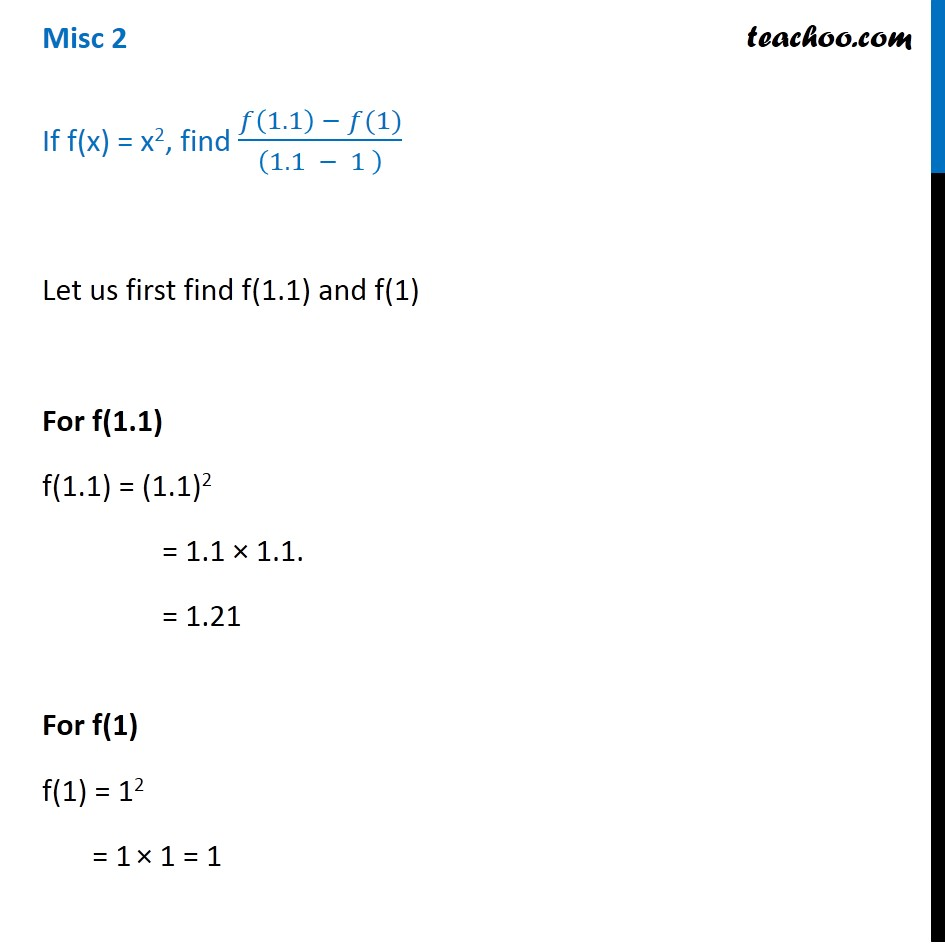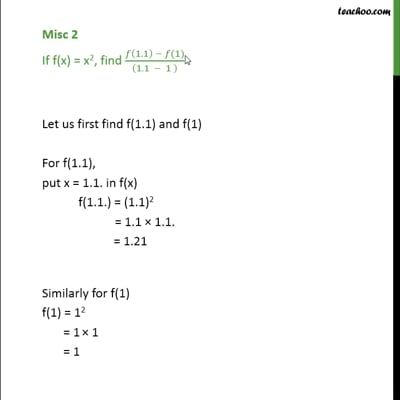Miscellaneous

Chapter 2 Class 11 Relations and Functions (Term 1)
Serial order wiseThis video is only available for Teachoo black users

### Transcript

Misc 2 If f(x) = x2, find (𝑓(1.1) − 𝑓(1))/((1.1 − 1 ) ) Let us first find f(1.1) and f(1) For f(1.1) f(1.1) = (1.1)2 = 1.1 × 1.1. = 1.21 For f(1) f(1) = 12 = 1 × 1 = 1 Now, (𝑓(1.1) − 𝑓(1))/((1.1 − 1 ) ) = ((1.1)^2 −(1)^2)/((1.1 − 1) ) = (1.21 − 1)/(1.1 − 1) = 0.21/0.1 = 2.1